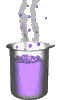Science class online
Chemistry and physics lessons

# Mechanics

Free online mechanics lesson for elementary school,  middle school and high school.Science class Chemistry Electricity Optics Mechanics Mechanics lessons Gravity - The solar system - motions of celestial bodies - Physical interactions - Effects of gravity - Weight of an object - Difference between weight and mass - Relationship between weight and mass  Energy - Energy basics - Potential energy - Kinetic energy - Mechanical energy - Translational Kinetic Energy - kinetic energy mass and speed

 _____________________________________________________   _____________________________________________________

## Gravity

### Mechanical energy

 1) What is mechanical energy ? The conversion of potential energy into kinetic energy for a falling object indicates a link between these two forms of energy. This link has led to define a new kind of energy: mechanical energy. Several symbols can be used: sometimes ME, TME or U Like other kinds of energy, mechanical energy can be expressed in joule. Definition: mechanical energy is the sum of kinetic energy and potential energy Mechanical energy = kinetic energy + potential energy ME = KE + PE ______________________________________ ______________________________________ 2) Conservation of mechanical energy If an object does not exchange energy with another object, its mechanical energy remains constant. If mechanical energy does not vary, it implies that every change of kinetic energy is simultaneously accompained by the opposite change of potential energy ( and reciprocally ). ______________________________________ ______________________________________
____________________________________

____________________________________